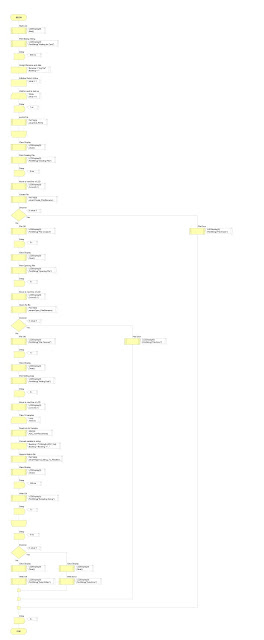## Monday, 6 July 2015

### PIC18F4455 ADC Sampling and Writing to SDCard FlowCode SimulationIn this project "ADC Sampling and Writing to SDCard" PIC series micro controller - PIC18F4455 has been used with Liquid Crystal Display (16X2) in Flowcode Simulation Software.As Source of ADC Metal POT (PotentioMeter ) has been used as the variables for desired results and final results are being written to SD Card FAT16 Type.

In this example we are using LCD to display the results of ADC calculation as seen in the examples code and videos.You can manipulate the POT while sampling is going on.As per concept of sampling POT should be kept at desired position to emulate it as sensor and then sampling needs to be done.

Here is the Flow code program:

ADC Related Sample Projects and Programming Codes
Here is the complete list of projects:

Tutorials for Flow Code Programming and Simulation

We are doing 10 Samples per cycle.In video you will be able to see step-wise execution of program and their effect on LCD in panel which is by default in the software .

Flow code Simulation and how to do Video Tutorial:

Little Introduction:An analog-to-digital converter (ADC, A/D, or A to D) is a device that converts a continuous physical quantity (usually voltage) to a digital number that represents the quantity's amplitude.The conversion involves quantization of the input, so it necessarily introduces a small amount of error. Instead of doing a single conversion, an ADC often performs the conversions ("samples" the input) periodically.

The result is a sequence of digital values that have been converted from a continuous-time and continuous-amplitude analog signal to a discrete-time and discrete-amplitude digital signal.An ADC is defined by its bandwidth (the range of frequencies it can measure) and its signal to noise ratio (how accurately it can measure a signal relative to the noise it introduces). The actual bandwidth of an ADC is characterized primarily by its sampling rate, and to a lesser extent by how it handles errors such as aliasing.

The dynamic range of an ADC is influenced by many factors, including the resolution (the number of output levels it can quantize a signal to), linearity and accuracy (how well the quantization levels match the true analog signal) and jitters (small timing errors that introduce additional noise). The dynamic range of an ADC is often summarized in terms of its effective number of bits (ENOB), the number of bits of each measure it returns that are on average not noise. An ideal ADC has an ENOB equal to its resolution. ADCs are chosen to match the bandwidth and required signal to noise ratio of the signal to be quantized. If an ADC operates at a sampling rate greater than twice the bandwidth of the signal, then perfect reconstruction is possible given an ideal ADC and neglecting quantization error. The presence of quantization error limits the dynamic range of even an ideal ADC, however, if the dynamic range of the ADC exceeds that of the input signal, its effects may be neglected resulting in an essentially perfect digital representation of the input signal.